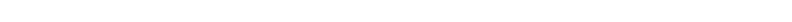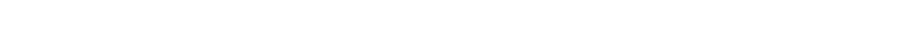For the Students of Hindu Vedic Astrology by Dr. A. Shanker

## Recent Posts## 20140422

Part 2

(Suppose a planet has six years as the period of its Period (main) and there is a planet located with it in the same sign, but not in the stipulated houses, their respective portions will be denoted by the fractions 1/1 and 1/2. Each multiplied by 2, the denominator gives 2 and 1. Hence the main Period lord will have 2/3rd and the sub-period lord 1/3rd of the whole period of the Period lord.

ii) If there is a planet in the 5th or 9th from the Period lord and there are no planets in the other stipulated houses, the fractions showing the respective shares of Period lord and the other planet would be 1/1 and 1/3 multiplied by denominator 3, the proportion will be 3 and 1 i.e. 3/4th and 1/4th of the total period of the main Period lord.

iii) If there is planet in the 4th or 8th from the Period lord and none in the other stipulated houses, the proportion will be indicated by fractions 1/1 and 1/4 which means 4 to 1, i.e. 4/5th and 1/5th respectively of the total period of the Period lord.

iv) If there is a planet located in 7th from the Period lord and none in the other stipulated houses, the proportion as between main and sub period lord will be 1/1 and 1/7 i.e. 7 to 1 or 7/8th and 1/8th respectively.

v) If there is a planet with the Period lord and also in 5th or 9th from it but none in 4th, 8th or 7th from it, the proportion would be 1/1, 1/2 and 1/3 which gives 6, 3 and 2 out of 11, hence the Period of the three planets, i.e. the main Period, lord, one with Period lord and one in the trine to Period lord would be 6/11th, 3/11th and 2/11th respectively of the total period of the Period lord.

vi) If a planet is with the Period lord and one in 4th or 8th from it, but none in trine from it then the proportion would be indicated by fractions 1/1, 1/2 and 1/4 i.e. 4, 2 and 1 or 4/7th, 2/7th and 1/7th respectively of the total period of Period lord.

vii) If a planet is with the Period lord and also one in 7th from it, but none in other stipulated house, the proportion would be denoted by 1/1, 1/2, 1/7 i.e. 14, 7 and 2 or 14/23rd, 7/23rd and 2/23rd of the total period of the main Period lord.

viii) If there are planets in trines from the Period lord and in none of the other stipulated houses, the proportion would be 1/1, 1/3 and 1/3, i.e. 3, 1, 1 or 3/5th, 1/5th and 1/5th respectively for main Period lord etc.

ix) If a planet is in one of the trines from the Period lord and also one in 4th or 8th from it, and none in the other stipulated houses, the proportion would be denoted by the fraction 1/1, 1/3, 1/4, or 12, 4 and 3 i.e. 12/19th, 4/19th and 3/19th of the total period of the main Period lord.

x) If a planet is in trine from the Period lord and also one in 7th from it, but none in the other stipulated houses, the proportion would be denoted by the fractions 1/1, 1/3 and 1/7 i.e. 21, 7 and 3 or 21/31, 7/31 and 3/31 of he whole period of the Period lord.

xi) If planets are in 4th and 8th from the Period lord, but none in other stipulated houses, the proportion would be denoted by the fractions 1/1, 1/4 and 1/4 i.e. 4, 1 and 1 or 4/6th, 1/6th and 1/6th respectively for the main Period lord and the other planets.

xii) If a planet is located in 4th, 8th and 7th from the Period lord, but not in the remaining stipulated houses, the proportion of sub-periods would be indicated by fractions 1/1, 1/4 and 1/7, i.e. 28, 7 and 4 i.e. 28/39, 7/39 and 4/39 respectively for the Period lord and the other planets.

xiii) If a planet is with the main Period lord and also one in each of the trines, the proportion of the sub-periods would be denoted by the fractions 1/1, 1/2, 1/3 and 1/3 i.e. 6, 3, 2 and 2, or 6/13, 3/13, 2/13 and 1/13 respectively for the Period lord and others. In this manner the sub-periods of the main Period lord and the sub-periods lord should be worked out for remaining positions of planets in relation to the Period lord).

The Period of the most powerful planet occupying at the time of birth the exaltation degree of its exaltation sign, is known as Sampoorna. The Period of a weak planet occupying the depression degree of its depression sign is known as Rikta. The Period of a planet occupying an inimical Navamsa and also the debilitation degree of its depression sign is known as Anishta.

The Period of a planet which quitting the exaltation degree moves towards the depression sign is known as Avarohini- Such Period periods produce evil.; while so moving, if the planet occupy a friendly or an exaltation Navamsa, his Period is known as Madhyama. Again, the Period of a planet which quitting the depression degree moves towards its exaltation sign is known as Arohini-Such Period periods produce prosperity.; while so moving, if the planet occupy an inimical or a depression Navamsa, his Period is known as Adhama.

Profile and Dr. Adawal’s Astro Channel

Dr. Adawal’s approved articles published on Articlesbase.com

Dr. Adawal’s exclusive articles on docstoc.com